Iteration function in Microsoft Excel

In this article, we are going to learn about Iterative. What is Iterative and how we can use iteration in Microsoft Excel.

Definition of Iteration:-

It is the repeated recalculation of a worksheet until a specific numeric condition is met. Excel cannot automatically calculate a formula that refers to the cell — either directly or indirectly — that contains the formula. This is called a circular reference. If a formula refers back to one of its own cells, you must determine how many times the formula should recalculate. Circular references can iterate indefinitely. However, you can control the the maximum number of iterations and the amount of acceptable change

Before applying the iteration formula in Excel, we have to add iterative option in Excel. To add the iterative option follow below steps-

• Go to file option.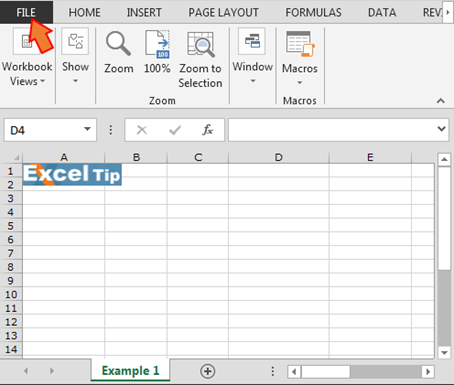• Click on Options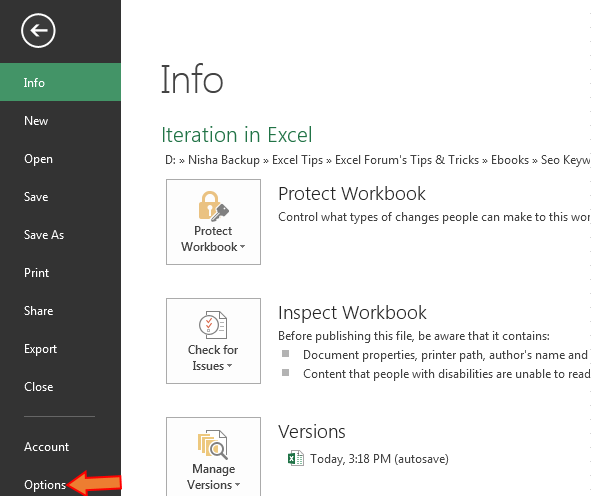• Excel Options dialog box will appear.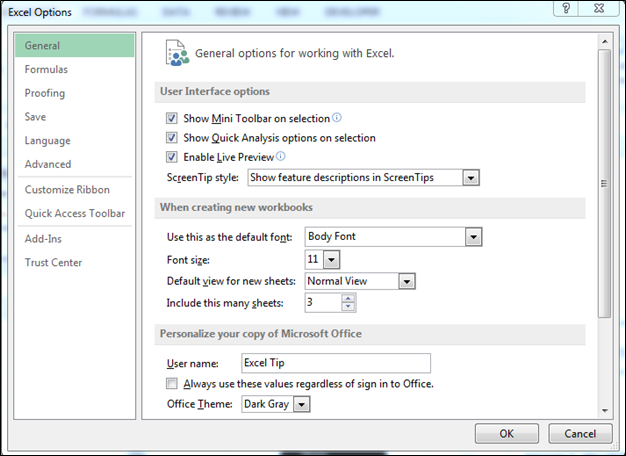• Click on Formulas option.
• Check the option of Enable Iterative calculations.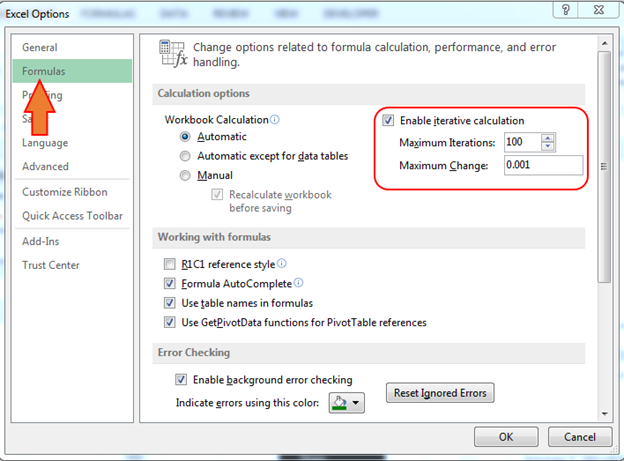• Click on ok.
• In Excel, Maximum iteration is 100 and Maximum change is 0.001, it is a default setting in Excel. Usually, Excel reaches sufficiently accurate results in as few as three iterations.

Circular references occur when a cell refers either directly or indirectly to the cell in which formula is stored.

Let’s understand with the examples the applications of Iteration in Microsoft Excel.

In this example, a Value-added tax (VAT) gross-up formula is in cell B1 (the formula is =B3-B2).
1. In cell A2, enter the VAT percent (%) 0.20.
2. In cell B2, insert the formula =A2*B1 to calculate the VAT amount.
3. Click OK to dismiss the circular reference warning.
4. In cell B3, enter 100. A Circular Reference is created in cell B1. The calculation of the amount without VAT is conditioned upon the calculation of VAT in cell B2.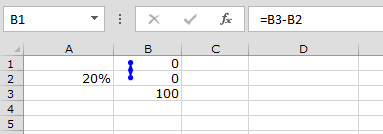Is this way, we use Iterative formula in Microsoft Excel.